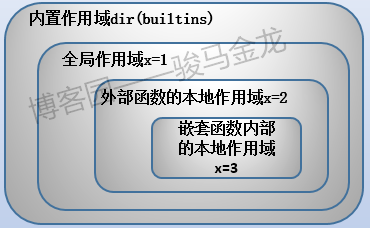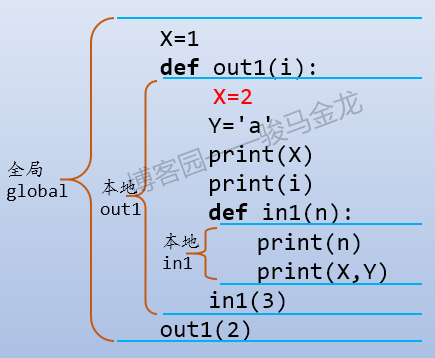设为首页 加入收藏
TOP
Python3基础-Python作用域详述(一)
2018-11-08 12:13:27 】 浏览:394
 Tags：Python3 基础 -Python 作用 详述

# Python作用域详述

python的变量作用域的规则非常简单，可以说是所有语言中最直观、最容易理解的作用域。

``````x=1
def f():
x=3
g()
print("f:",x)   # 3

def g():
print("g:",x)   # 1

f()
print("main:",x)    # 1``````

## python作用域规则简介• 内置作用域是预先定义好的，在`__builtins__`模块中。这些名称主要是一些关键字，例如open、range、quit等
• 全局作用域是文件级别的，或者说是模块级别的，每个py文件中处于顶层的变量都是全局作用域范围内的变量
• 本地作用域是函数内部属于本函数的作用范围，因为函数可以嵌套函数，嵌套的内层函数有自身的内层范围
• 嵌套函数的本地作用域是属于内层函数的范围，不属于外层

``````X=1
def out1(i):
X=2
Y='a'
print(X)
print(i)
def in1(n):
print(n)
print(X,Y)
in1(3)
out1(2)``````## 内置作用域

``````>>> import builtins
>>> dir(builtins)
['ArithmeticError', 'AssertionError', 'AttributeError', 'BaseException', 'BlockingIOError', 'BrokenPipeError', 'BufferError', 'BytesWarning', ...............
'range', 'repr', 'reversed', 'round', 'set', 'setattr', 'slice', 'sorted', 'staticmethod', 'str', 'sum', 'super', 'tuple', 'type', 'vars', 'zip']``````

## 变量掩盖和修改规则

``````x=3
def g():
print(x)  # 引用全局变量x``````

``````x=3
def g():
x=2   # 定义并赋值本地变量x
print(x)  # 引用本地变量x``````

python是一种解释性语言，读一行解释一行，读了下一行就忘记前一行(详细见下文)。所以在使用变量之前必须先进行变量的定义(声明)

``````def g():
print(x)
x=3
g()``````

``````UnboundLocalError: local variable 'x' referenced
before assignment``````

``````x=1
def g():
print(x)
x=2
g()``````

``````x=1
def g():
x=2
print(x)
g()``````

``````g()
def g():
x=2
print(x)``````

``NameError: name 'g' is not defined``

``````x=1
def f():
x=3
g()
print("f:",x)   # 3

def g():
print("g:",x)   # 1

f()
print("main:",x)    # 1``````

】【打印繁体】【投稿】【收藏】 【推荐】【举报】【评论】 【关闭】 【返回顶部array(4) { ["type"]=> int(8) ["message"]=> string(24) "Undefined variable: jobs" ["file"]=> string(32) "/mnt/wp/cppentry/do/bencandy.php" ["line"]=> int(214) }